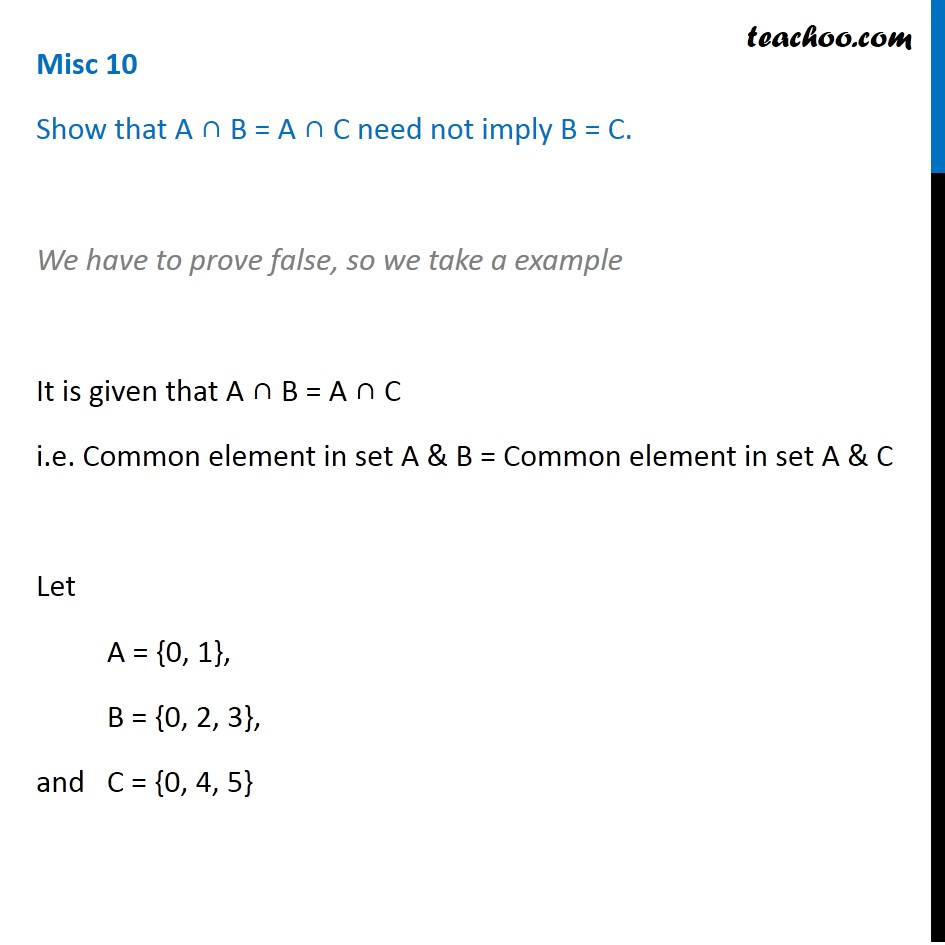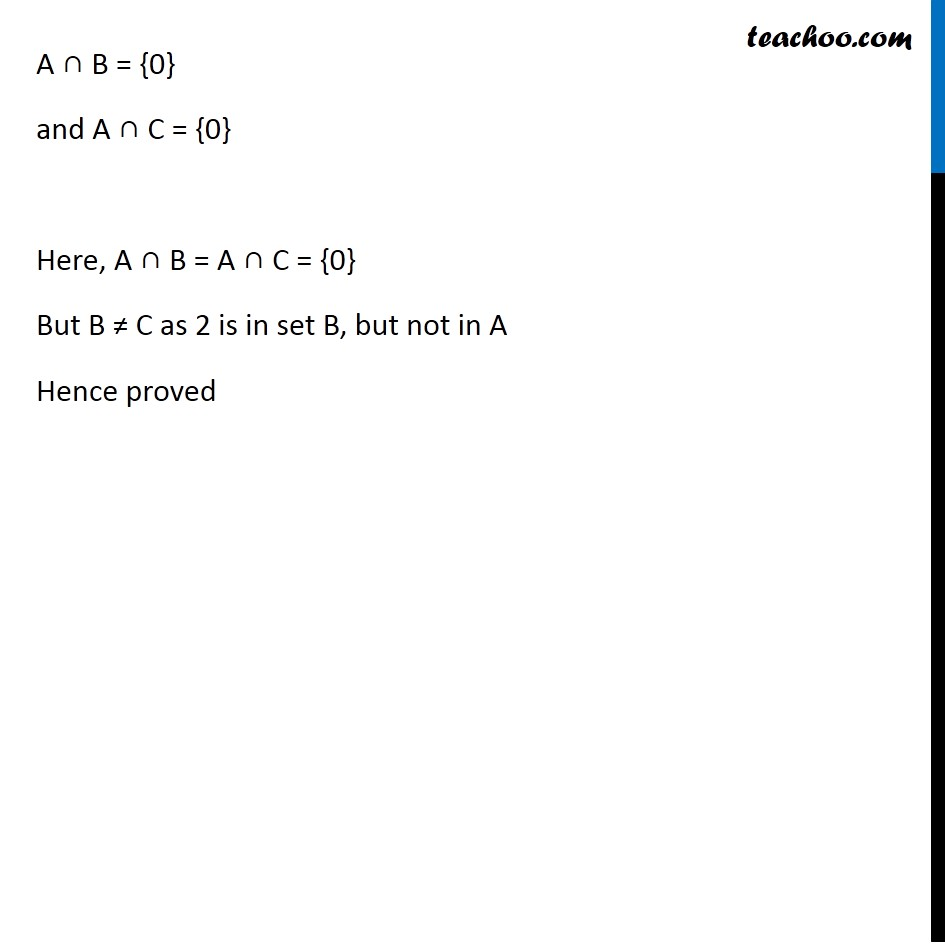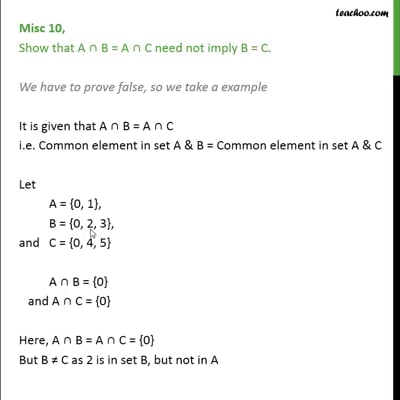Proof - Using properties of sets

Chapter 1 Class 11 Sets
Concept wiseThis video is only available for Teachoo black users

Introducing your new favourite teacher - Teachoo Black, at only ₹83 per month

### Transcript

Misc 10 Show that A ∩ B = A ∩ C need not imply B = C. We have to prove false, so we take a example It is given that A ∩ B = A ∩ C i.e. Common element in set A & B = Common element in set A & C Let A = {0, 1}, B = {0, 2, 3}, and C = {0, 4, 5} A ∩ B = {0} and A ∩ C = {0} Here, A ∩ B = A ∩ C = {0} But B ≠ C as 2 is in set B, but not in A Hence proved Misc 11 Let A and B be sets. If A ∩ X = B ∩ X = ∅ and A ∪ X = B ∪ X for some set X, show that A = B. (Hints: A = A ∩ (A ∪ X), B = B ∩ (B ∪ X) and use distributive law) Given: Let A and B be two sets such that A ∩ X = B ∩ X = ∅ and A ∪ X = B ∪ X for some set X. To prove: A = B Proof: Let A = A ∩ (A ∪ X) A = A ∩ (B ∪ X) (Given A ∪ X = B ∪ X) Let A = A ∩ (A ∪ X) Given A ∪ X = B ∪ X A = A ∩ (B ∪ X) Using distributive law : A ∩ (B ∪ C)= (A ∩ B) ∪ (A ∩ C) = (A ∩ B) ∪ (A ∩ X) As A ∩ X = ∅ given = (A ∩ B) ∪ ∅ A = A ∩ B Let B = B ∩ (B ∪ X) Given A ∪ X = B ∪ X B = B ∩ (A ∪ X) Using distributive law: A ∪ (B ∩ C)= (A ∩ B) ∪ (A ∩ C) = (B ∩ A) ∪ (B ∩ X) As B ∩ X = Φ = (B ∩ A) ∪ Φ B = B ∩ A B = A ∩ B From (1) and (2), A = A ∩ B & B = A ∩ B ∴ A = B Hence proved